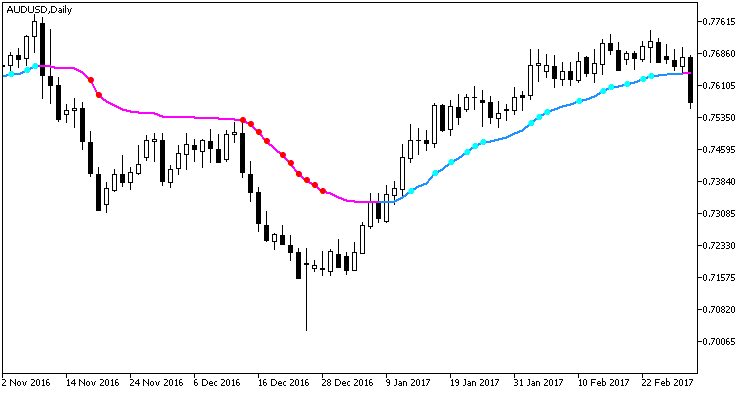# EMAVFS_StDev – indicator for MetaTrader 5

• A+
category：MT5 INDICATORS

The EMAVFS indicator with additional trend strength indication using colored dots based on the standard deviation algorithm.

If the standard deviation of the EMAVFS indicator is between the dK1 and dK2 parameter values, then a small colored dot appears on the moving average. Its color corresponds to the current trend direction.

```input double dK1=1.5; // Square-law filter coefficient 1
input double dK2=2.5;  // Square-law filter coefficient 2
```

If the standard deviation becomes higher than the dK2 input parameter value, then the dot size increases. Thus, we get three levels of trend strength indication.

1. Weak — no dots;
2. Medium — small colored dots;
3. Strong — big colored dots.Fig.1. Indicator EMAVFS_StDev